An Allen-Bradley SLC500 programmable logic controller (PLC) uses a 16-bit analog-to-digital converter ( in its model 1746-NI4 ) in analog input card to convert 4-20 mA signals into digital number values ranging from 3277 (at 4 mA) to 16384 (at 20 mA).

However, these raw numbers from the PLC’s analog card must be mathematically scaled inside the PLC to represent real-world units of measurement, in this case 0 to 700 GPM of flow.

Formulate a scaling equation to program into the PLC so that 4 mA of current registers as 0 GPM, and 20 mA of current registers as 700 GPM.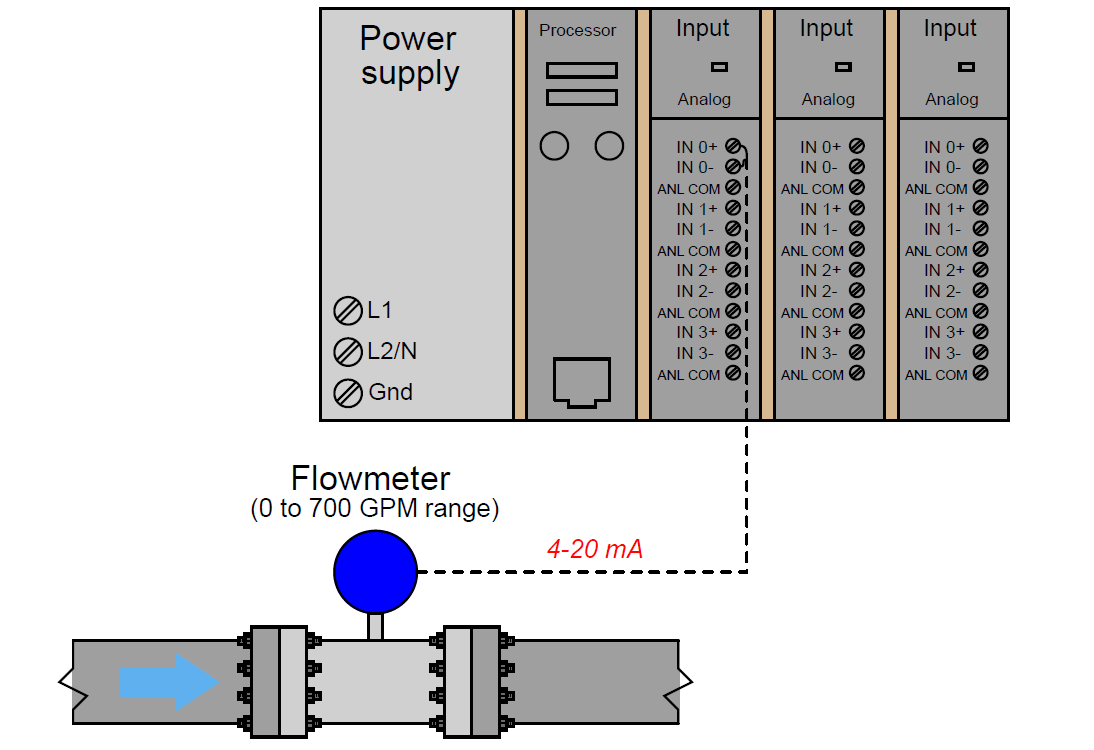We are already given the raw number values from the analog card’s analog-to-digital converter (ADC) circuit for 4 mA and 20 mA: 3277 and 16384, respectively.

These values define the domain of our linear graph: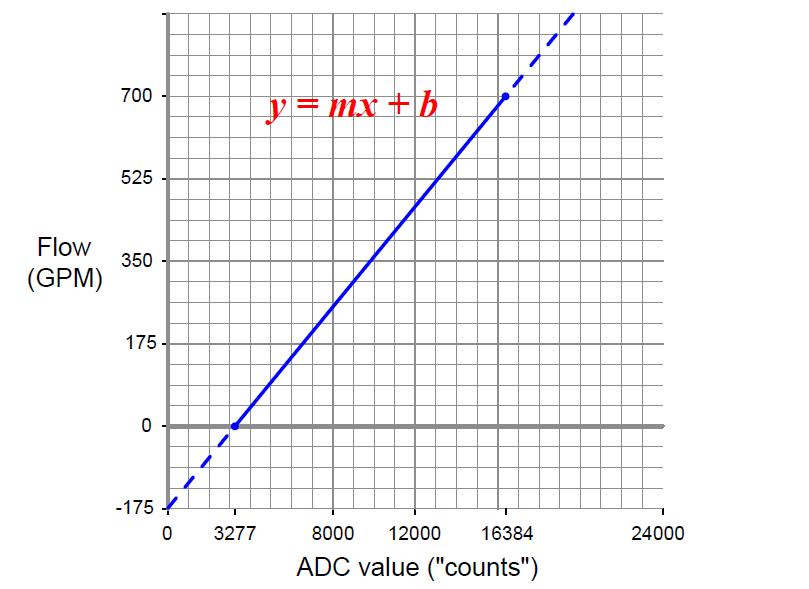Calculating and substituting the slope (m) value for this equation, using the full rise-over-run of the linear function: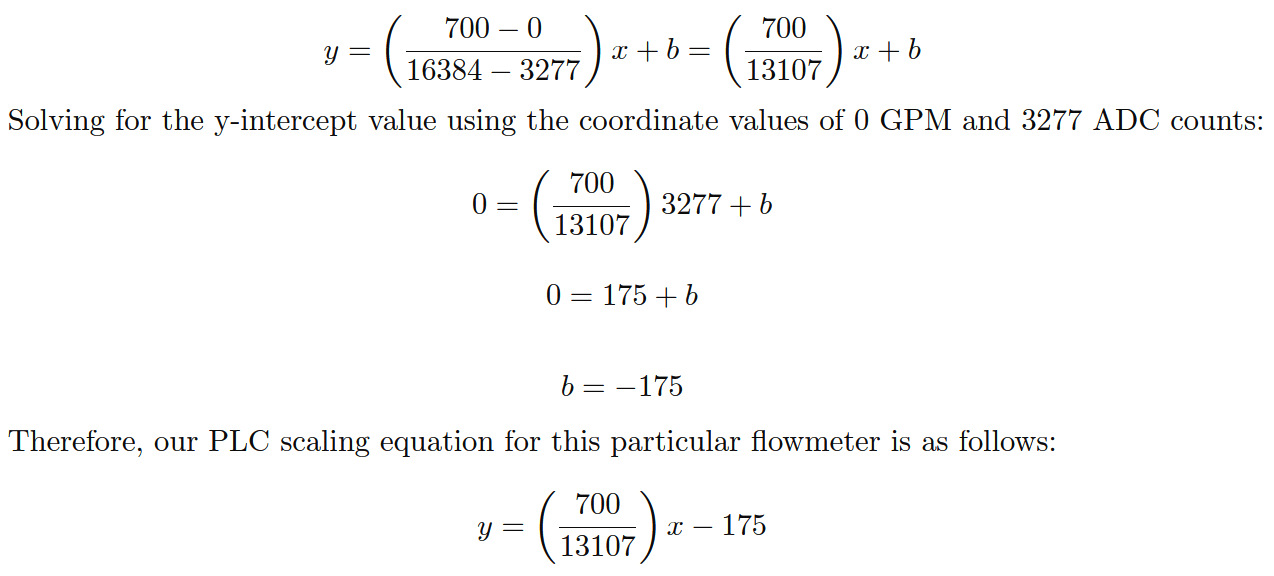Also Read : PLC Raw Count Calculation

This type of scaling calculation is so common in PLC applications that Allen-Bradley has provided a special SCL (“scale”) instruction just for this purpose.

Instead of “slope” (m) and “intercept” (b), the instruction prompts the human programmer to enter “rate” and “offset” values, respectively.

Furthermore, the rate in Allen-Bradley’s SCL instruction is expressed as the numerator of a fraction where the denominator is fixed at 10000, allowing fractional (less than one) slope values to be specified using integer numbers.

Aside from these details, the concept is exactly the same. Expressing our slope of 700/13107 as a fraction with 10000 as the denominator is a simple matter of solving for the numerator using cross-multiplication and division: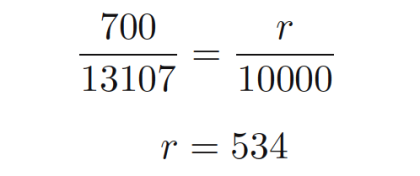Thus, the SCL instruction would be configured as follows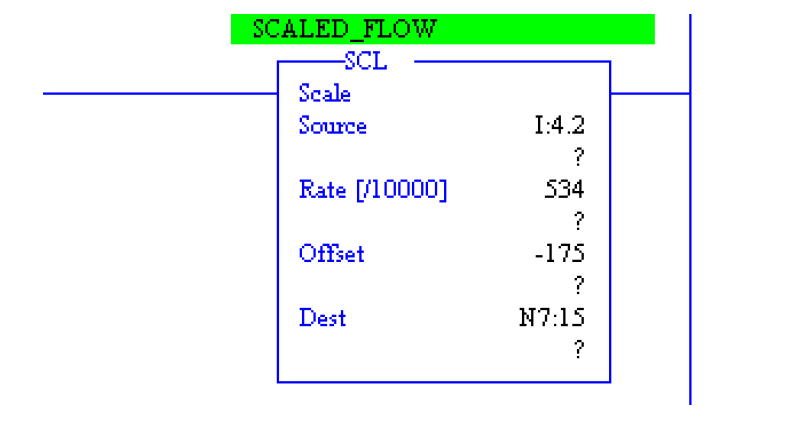Also Read : 4-20mA Conversion Formulas

Credits : by Tony R. Kuphaldt – Creative Commons Attribution 4.0 License

#### PLC Program for Flow TotalizerAugust 11, 2017 at 10:50 am

thank youJuly 26, 2019 at 7:42 pm

ThanksJuly 26, 2019 at 8:24 pm

Always see the signal from control systems point of view.

Example-